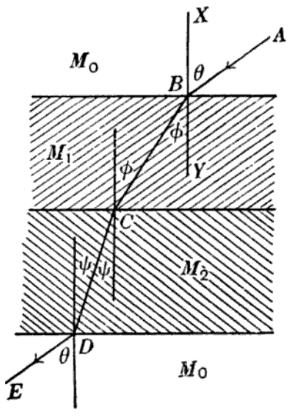# State laws of refraction

The incident ray AB and the refracted ray BC and the perpendicular of B to the surface separating the two media Mo and M1 lie in the same plane.The angle of incidence is the angle between the incident ray and the normal, denoted as ‘i’. The angle of refraction is the angle between the refracted ray and the normal, denoted as ‘r’. Laws of refraction state that:

• The incident ray, reflected ray and the normal, to the interface of any two given mediums, all lie in the same plane.
• The ratio of the sine of the angle of incidence and sine of the angle of refraction is constant.  This is also known as Snell’s law of refraction(200)(26)